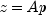JMSLTM Numerical Library 7.2.0
com.imsl.math

## Interface GenMinRes.Function

• All Known Subinterfaces:
GenMinRes.Preconditioner
Enclosing class:
GenMinRes

`public static interface GenMinRes.Function`
Public interface for the user supplied function to `GenMinRes`.
• ### Method Summary

Methods
Modifier and Type Method and Description
`void` ```amultp(double[] p, double[] z)```
Used to computewhere `A` is the matrix of coefficients to solve and `p` and `z` are arrays of length `n`, the order of matrix `A`.
• ### Method Detail

• #### amultp

```void amultp(double[] p,
double[] z)```
Used to computewhere `A` is the matrix of coefficients to solve and `p` and `z` are arrays of length `n`, the order of matrix `A`.
Parameters:
`p` - an input `double` array of length `n` generated during the implementation of the `solve` method.
`z` - an output `double` array of length `n`.
JMSLTM Numerical Library 7.2.0